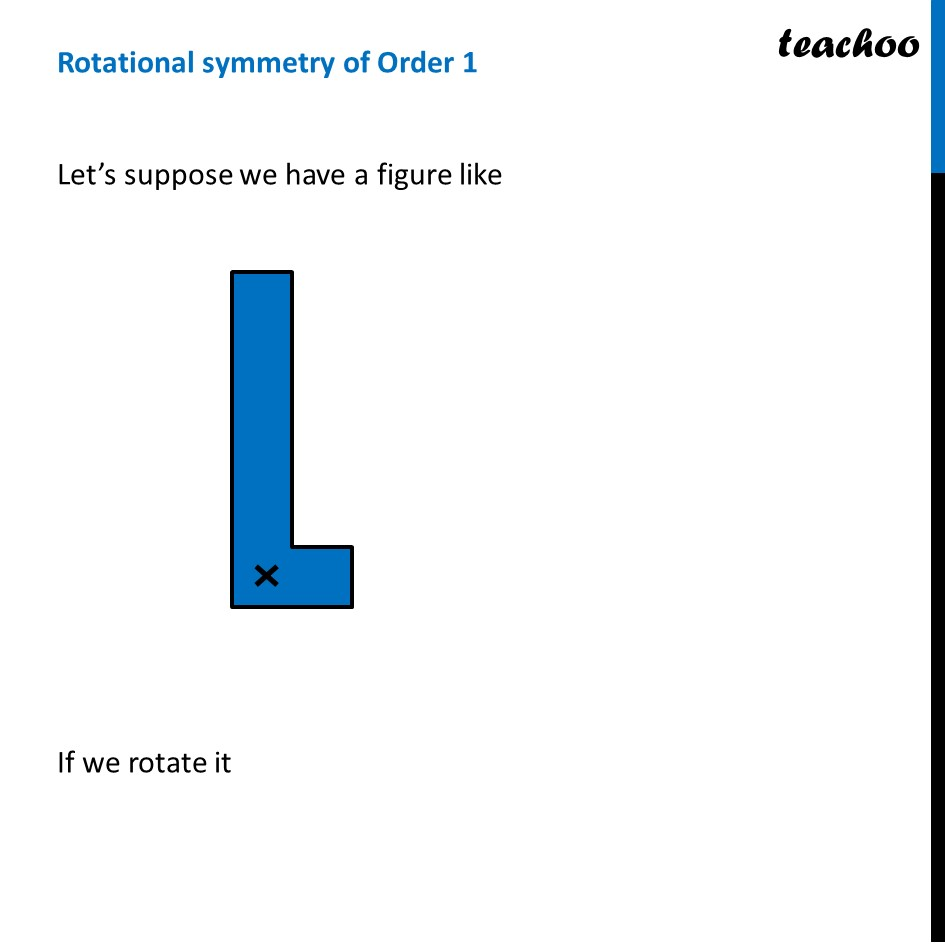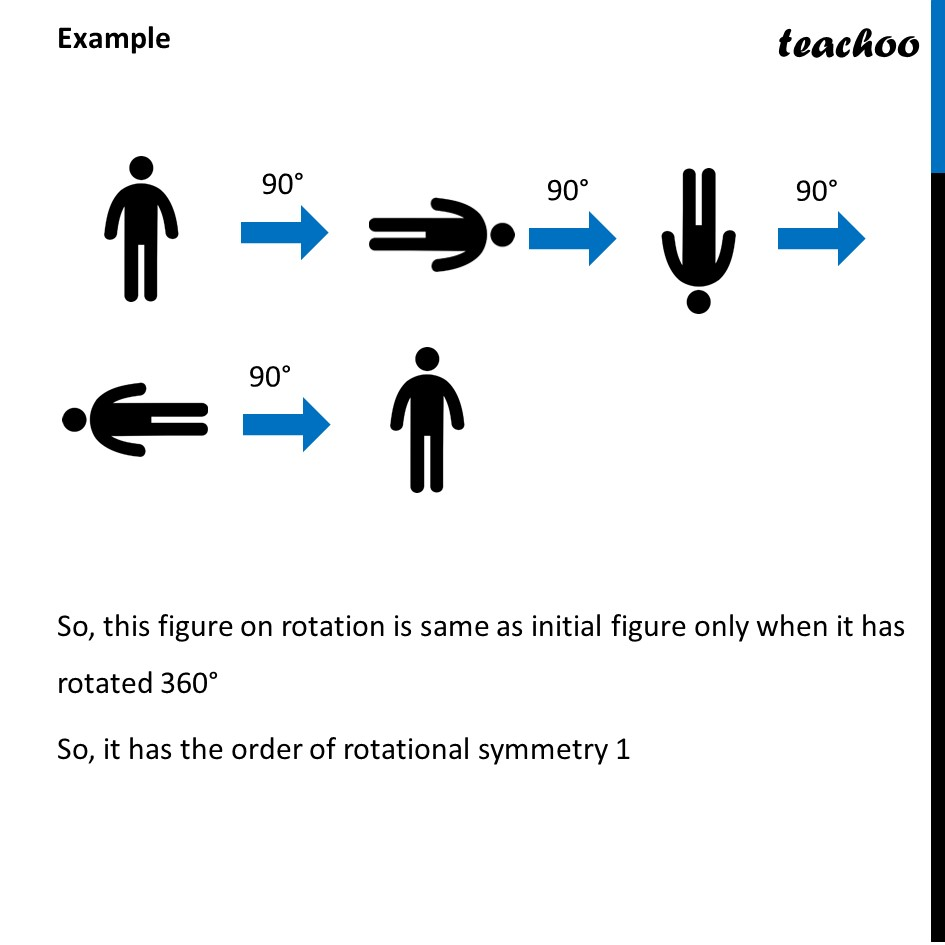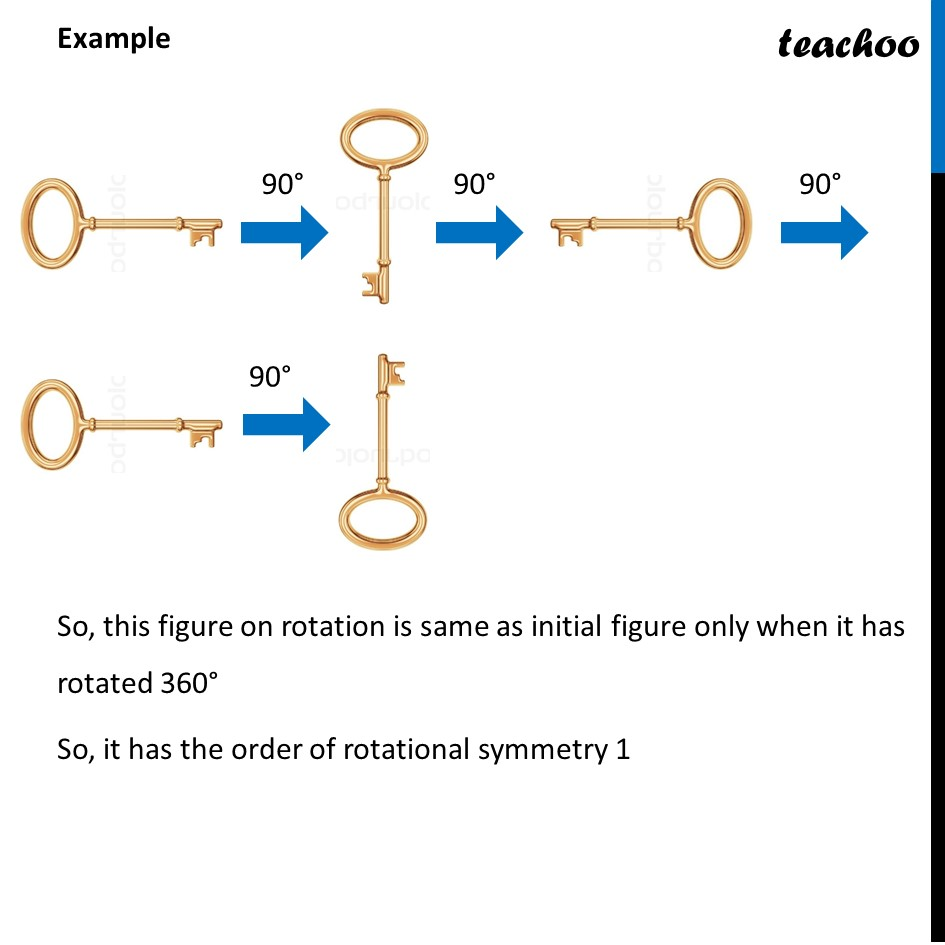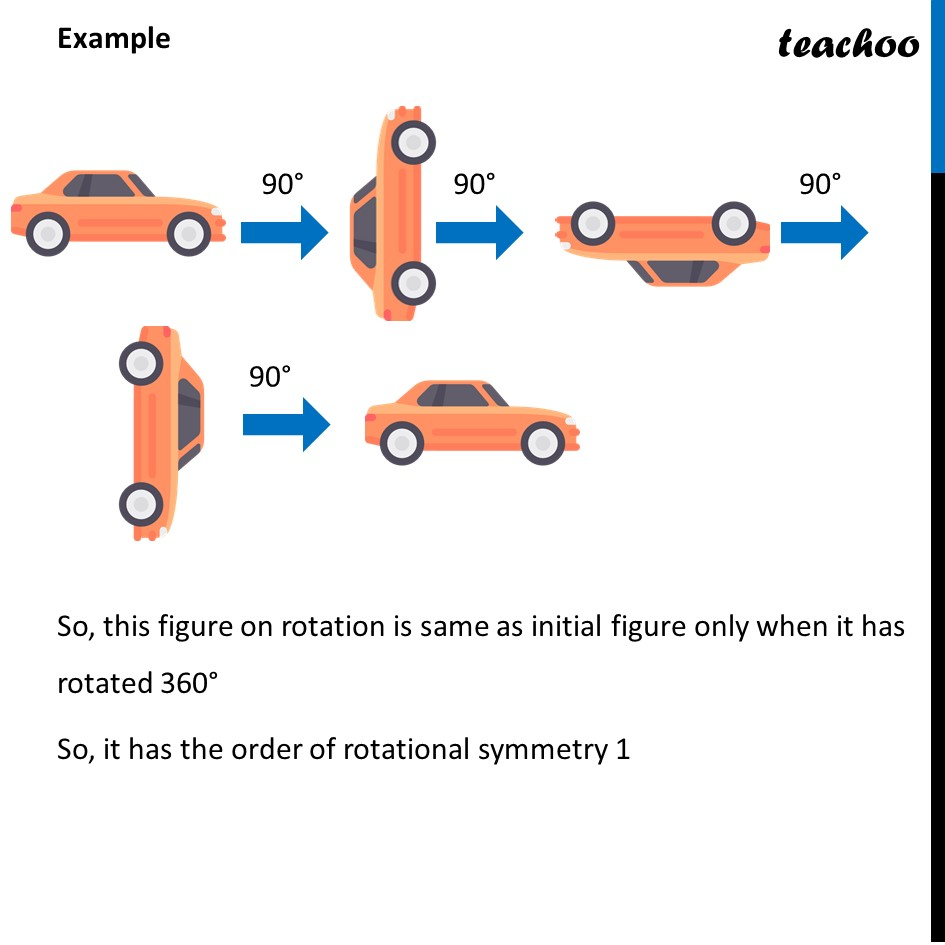Lines and Rotational symmetry (Both)

Chapter 12 Class 7 Symmetry
Concept wiseLearn in your speed, with individual attention - Teachoo Maths 1-on-1 Class

### Transcript

Rotational symmetry of Order 1Let’s suppose we have a figure like If we rotate it So, this figure on rotation is same as initial figure Only when it has rotated 360° So, it has order of rotational symmetry 1 Every object has order of rotational symmetry 1 Example So, this figure on rotation is same as initial figure only when it has rotated 360° So, it has the order of rotational symmetry 1 Example So, this figure on rotation is same as initial figure only when it has rotated 360° So, it has the order of rotational symmetry 1 Example So, this figure on rotation is same as initial figure only when it has rotated 360° So, it has the order of rotational symmetry 1 Example So, this figure on rotation is same as initial figure only when it has rotated 360° So, it has the order of rotational symmetry 1